Atmos. Chem. Phys., 19, 6737–6747, 2019
https://doi.org/10.5194/acp-19-6737-2019
Atmos. Chem. Phys., 19, 6737–6747, 2019
https://doi.org/10.5194/acp-19-6737-2019

Research article 21 May 2019

Research article | 21 May 2019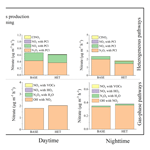# Modeling the impact of heterogeneous reactions of chlorine on summertime nitrate formation in Beijing, China

Modeling the impact of heterogeneous reactions of chlorine on summertime nitrate formation in Beijing, China
Xionghui Qiu1,2, Qi Ying3, Shuxiao Wang1,2, Lei Duan1,2, Jian Zhao4, Jia Xing1,2, Dian Ding1,2, Yele Sun4, Baoxian Liu5, Aijun Shi6, Xiao Yan6, Qingcheng Xu1,2, and Jiming Hao1,2 Xionghui Qiu et al.
• 1State Key Joint Laboratory of Environmental Simulation and Pollution Control, School of Environment, Tsinghua University, Beijing 100084, China
• 2State Environmental Protection Key Laboratory of Sources and Control of Air Pollution Complex, School of Environment, Tsinghua University, Beijing 100084, China
• 3Zachry Department of Civil Engineering, Texas A&M University, College Station, Texas 77843-3138, USA
• 4State Key Laboratory of Atmospheric Boundary Layer Physics and Atmospheric Chemistry, Institute of Atmospheric Physics, Chinese Academy of Sciences, Beijing 100029, China
• 5Beijing Environmental Monitoring Center, Beijing 100048, China
• 6Beijing Municipal Research Institute of Environmental Protection, Beijing 100037, China

Correspondence: Qi Ying (qying@civil.tamu.edu) and Shuxiao Wang (shxwang@tsinghua.edu.cn)

Abstract

Comprehensive chlorine heterogeneous chemistry is incorporated into the Community Multiscale Air Quality (CMAQ) model to evaluate the impact of chlorine-related heterogeneous reaction on diurnal and nocturnal nitrate formation and quantify the nitrate formation from gas-to-particle partitioning of HNO3 and from different heterogeneous pathways. The results show that these heterogeneous reactions increase the atmospheric Cl2 and ClNO2 level (∼ 100 %), which further affects the nitrate formation. Sensitivity analyses of uptake coefficients show that the empirical uptake coefficient for the O3 heterogeneous reaction with chlorinated particles may lead to the large uncertainties in the predicted Cl2 and nitrate concentrations. The N2O5 uptake coefficient with particulate Cl concentration dependence performs better in capturing the concentration of ClNO2 and nocturnal nitrate concentration. The reaction of OH and NO2 in the daytime increases the nitrate by ∼15 % when the heterogeneous chlorine chemistry is incorporated, resulting in more nitrate formation from HNO3 gas-to-particle partitioning. By contrast, the contribution of the heterogeneous reaction of N2O5 to nitrate concentrations decreases by about 27 % in the nighttime, when its reactions with chlorinated particles are considered. However, the generated gas-phase ClNO2 from the heterogeneous reaction of N2O5 and chlorine-containing particles further reacts with the particle surface to increase the nitrate by 6 %. In general, this study highlights the potential of significant underestimation of daytime concentrations and overestimation of nighttime nitrate concentrations for chemical transport models without proper chlorine chemistry in the gas and particle phases.

Share
1 Introduction

In recent years, nitrate has become the primary component of PM2.5 (particulate matter with an aerodynamic diameter less than 2.5 µm) in Beijing, with sustained and rapid reduction of SO2 and primary particulate matter emissions (Ma et al., 2018; Li et al., 2018; Wen et al., 2018). Observations showed that the relative contributions of secondary nitrate in PM2.5 could reach up to approximately 50 % during some severe haze pollution days (Li et al., 2018). The mechanism of secondary nitrate formation can be summarized as two major pathways. (1) Gas-to-particle partitioning of HNO3 happens mostly in the daytime. The reaction of OH with NO2 produces gaseous HNO3, which subsequently partitions into the particle phase. The existence of NH3 or basic particles enhances this process by NH3${\mathrm{NH}}_{\mathrm{4}}^{+}$ gas–particle equilibrium (Kleeman et al., 2005; Seinfeld and Pandis, 2006). (2) Hydrolysis of N2O5 is more important during the nighttime, when N2O5 forms from the reactions of NO2, O3 and NO3 and hydrolyzes to produce particulate nitrate. They can be summarized as Reactions (R1)–(R5) (Brown and Stutz, 2012):

$\begin{array}{}\text{(R1)}& & \mathrm{OH}+{\mathrm{NO}}_{\mathrm{2}}\to {\mathrm{HNO}}_{\mathrm{3}},\text{(R2)}& & {\mathrm{HNO}}_{\mathrm{3}}+{\mathrm{NH}}_{\mathrm{3}}\to {\mathrm{NH}}_{\mathrm{4}}^{+}+{\mathrm{NO}}_{\mathrm{3}}^{-},\text{(R3)}& & {\mathrm{NO}}_{\mathrm{2}}+{\mathrm{O}}_{\mathrm{3}}\to {\mathrm{NO}}_{\mathrm{3}}+{\mathrm{O}}_{\mathrm{2}},\text{(R4)}& & {\mathrm{NO}}_{\mathrm{3}}+{\mathrm{NO}}_{\mathrm{2}}↔{\mathrm{N}}_{\mathrm{2}}{\mathrm{O}}_{\mathrm{5}},\text{(R5)}& & {\mathrm{N}}_{\mathrm{2}}{\mathrm{O}}_{\mathrm{5}}+{\mathrm{H}}_{\mathrm{2}}\mathrm{O}\left(\mathrm{aq}\right)\to \mathrm{2}{\mathrm{H}}^{+}+\mathrm{2}{\mathrm{NO}}_{\mathrm{3}}^{-}.\end{array}$

In addition to Reactions (R1) and (R5), gas-phase reactions of NO3 with HO2 and VOCs, N2O5 with water vapor (Tuazon et al., 1983), and the heterogeneous reaction of NO2 with the water-containing particle (Goodman et al., 1999) produce HNO3 or nitrate as well. Theses reactions are listed in Table 2 as Reactions (R8), (R9) and (R10).

However, chemistry transport models (CTMs) incorporated with these mechanisms still cannot accurately capture the spatiotemporal distributions of nitrate in some studies in polluted northern China. For example, Chang et al. (2018) showed that the simulated nitrate concentrations derived from the default Community Multiscale Air Quality (CMAQ; version 5.0.2) were significantly higher than the observations in summer at two sites adjacent to Beijing. Fu et al. (2016) also found that the default CMAQ (version 5.0.1) overestimated the simulated nitrate concentrations in the Beijing–Tianjin–Hebei region.

In recent fields studies, it was found that high particulate chlorine emissions might have a significant impact on the oxidation capacity of the urban atmosphere and thus could affect nitrate concentrations. According to the field measurements in June 2017 in Beijing (Zhou et al., 2018), the 2 min averaged concentrations of reactive molecular chlorine (Cl2) and nitryl chloride (ClNO2) reached up to 1000 and 1200 pptv, respectively, during some severe air pollution periods in summer. The Cl2 concentrations were significantly higher than those observed in North American coastal cities affected by onshore flow and the lower atmosphere in the remote Arctic region (Spicer et al., 1998; Glasow, 2010; Liu et al., 2017). During these pollution events, the corresponding concentrations of N2O5 (2 min average) and nitrate (5 min average) rose from 40 pptv and 1 µg m−3 to 700 pptv and 5 µg m−3. To explain the high levels of ClNO2, some studies suggested that Reaction (R5) should be revised to account for ClNO2 production from the heterogeneous reaction of N2O5 on chloride-containing particles (CPs; Osthoff et al., 2008; Thornton et al., 2010), as shown in Reaction (R6):

$\begin{array}{ll}& {\mathrm{N}}_{\mathrm{2}}{\mathrm{O}}_{\mathrm{5}}+\left(\mathrm{1}-\mathit{\varphi }\right){\mathrm{H}}_{\mathrm{2}}\mathrm{O}+\mathit{\varphi }{\mathrm{Cl}}^{-}\to \mathit{\varphi }{\mathrm{ClNO}}_{\mathrm{2}}\\ \text{(R6)}& & +\left(\mathrm{2}-\mathit{\varphi }\right){\mathrm{NO}}_{\mathrm{3}}^{-},\end{array}$

where ϕ represents the molar yield of ClNO2. By incorporating this reaction into WRF-Chem, Li et al. (2016) found that the improved model performed better in matching the observed nitrate concentrations in Hong Kong during 15 November and 5 December 2013. However, ClNO2 could affect the formation of nitrate indirectly by increasing the atmospheric OH after a series of chemical reactions, which are briefly summarized into three steps: (1) the photolysis of ClNO2 produces chlorine radicals (Cl), (2) the reaction of Cl with VOCs produces the peroxy radical (HO2 and RO2), and (3) the increased HO2 and RO2 prompt the formation of OH through the HOx cycle and lead to increased HNO3 production (Young et al., 2014; Jobson et al., 1994). The overall impact of Reaction (R6) on nitrate remains to be investigated.

Another related but unresolved issue is the sources of the high concentrations of Cl2, which could not be explained by the N2O5 heterogeneous reaction with Cl and the subsequent reactions of ClNO2 in the gas phase. It has been reported that the reactions of gaseous O3, OH, HO2, ClNO2, hypochlorous acid (HOCl) and chlorine nitrate (ClONO2) with CPs can produce Cl2, which can subsequently photolyze to produce Cl (Knipping et al., 2000; George and Abbatt, 2010; Pratte and Rossi, 2006; Deiber et al., 2004; Faxon et al., 2015). However, these heterogeneous reactions in CPs are generally missing in most of the current CTMs, and it is unclear whether these reactions will be able to explain the observed Cl2 concentrations and the overall impact of these reactions on nitrate.

Previously, biomass burning, coal combustion and waste incineration were identified as the main sources of gaseous and particulate chlorine compounds in China from the International Global Atmospheric Chemistry program's Global Emissions Inventory Activity (GEIA) based on the year 1990 and a localized study by Fu et al. (2018) based on the year 2014 (Keene et al., 1999; Fu et al., 2018). However, recent source apportionment results of PM2.5 in Beijing showed that the contribution of coal combustion had extremely decreased from 22.4 % in 2014 to 3 % in 2017 with the replacement of natural gas (obtained from official website of the Beijing Municipal Bureau of Statistics, available at http://edu.bjstats.gov.cn/, last access: 5 May 2018). Another important source – cooking – has received attention with its increasing contribution to PM2.5 (accounting for 33 % of the residential sector; obtained from the official source apportionment analysis of PM2.5 in Beijing in 2017; see http://sthjj.beijing.gov.cn/, last access: 5 May 2018). Moreover, the high content of particulate sodium chloride was measured from the source characterization studies of PM2.5 released from the cooking activities (Zhang et al., 2016). Thus, it is necessary to compile an updated emission inventory for Beijing to include the emissions from cooking and other sources (coal burning, solid waste incineration, biomass burning, etc.) in order to explore the emissions of the chlorine species on atmospheric nitrate formation.

In this study, a CMAQ model with improved chlorine heterogeneous chemistry is applied to simulate summer nitrate concentration in Beijing. Sensitivity simulations are conducted to evaluate the contributions of HNO3 gas-to-particle partitioning and heterogonous production to aerosol nitrate. The results of this work can improve our understandings on nitrate formation and provide useful information on nitrate pollution control strategies in Beijing.

2 Emissions, chemical reactions and model description

## 2.1 Emissions

Generally, the conventional emission inventories of air pollutants in China only include the common chemical species, such as SO2, NOx, VOCs, PM2.5, PM10, NH3, BC and OC (Wang et al., 2014). Chloride compound emissions were not included. However, the emissions of chlorine species are vital for studying the chlorine chemical mechanism. Recently, the inorganic hydrogen chloride (HCl) and fine particulate chloride (PCl) emission inventories for the sectors of coal combustion, biomass burning and waste incineration were developed for the year 2014 (Qiu et al., 2016; Fu et al., 2018; Liu et al., 2018). However, gaseous chlorine emissions were not estimated in these studies. In addition, these studies did not account for the rapid decrease in coal consumption in recent years in Beijing, from 2000 Mt in 2014 to 490 Mt in 2017. More importantly, the cooking source, as one of the major contributors to particulate chlorine in Beijing, is not included in current chlorine emission inventories. Thus, a new emission inventory of reactive chlorine species, which includes HCl, Cl2 and PCl, was developed in this study for the year 2017.

The emission factor method (Eq. 1) is applied to calculate the emissions of these reactive chlorine species from coal combustion, biomass burning, municipal solid waste incineration and industrial processes:

$\begin{array}{}\text{(1)}& {E}_{i,j}={A}_{i}×{\mathrm{EF}}_{i,j},\end{array}$

where Ei,j represents the emission factor of pollutant j in sector i, A represents the activity data and EF represents the emission factor. The EF for PCl is estimated by ${\mathrm{EF}}_{i,\mathrm{PCl}}={\mathrm{EF}}_{i,{\mathrm{PM}}_{\mathrm{2.5}}}×{f}_{\mathrm{Cl},i}$, where fCl,i represents the mass fraction of PCl in primary PM2.5. Activity data are obtained from the Beijing Municipal Bureau of Statistics (available at http://tjj.beijing.gov.cn/, last access: 4 May 2018). The Cl2 emission factor for coal combustion is calculated based on the content of Cl in coal, which was measured by Deng et al. (2014). The PM2.5 emission factors and mass fractions of PCl in PM2.5 to calculate the emissions of Cl were described in detail by Fu et al. (2018). PCl in PM2.5 for coal combustion and biomass burning is taken as 1 % and 9.0 %, respectively, based on local measurements in Beijing.

Emissions of PCl from cooking, including contributions from commercial and household cooking, are estimated using Eq. (2):

$\begin{array}{ll}{E}_{\mathrm{PCl}}=& \phantom{\rule{0.125em}{0ex}}\left[{N}_{\mathrm{f}}×{V}_{\mathrm{f}}×{H}_{\mathrm{f}}×{\mathrm{EF}}_{\mathrm{f},\mathrm{PCl}}+{V}_{\mathrm{c}}×{H}_{\mathrm{c}}×{N}_{\mathrm{c}}\right\\ \text{(2)}& & ×n×{\mathrm{EF}}_{\mathrm{c},\mathrm{PCl}}×\left(\mathrm{1}-\mathit{\eta }\right)]×\mathrm{365},\end{array}$

where Nf is the number of households; Vf is the volume of exhaust gas from a household stove (2000 m3 h−1); Hf is the cooking time for a family (0.5 h d−1); EFf,PCl and EFc,PCl are the emission factors (kg m−3) of PCl for household and commercial cooking, respectively; Hc is the cooking time in a commercial cooking facility (6 h d−1); Nc is the number of restaurants, schools and government departments; Vc is the volume of exhaust gas from a commercial cooking stove (8000 m3 h−1); and n is the number of stoves for each unit, which is six for a restaurant and is calculated as one stove per 150 students for each school. η is the removal efficiency of fume scrubbers (30 %). EFc,PCl is the emission factor (kg m−3) of PCl in commercial cooking. These constants are all based on Wu et al. (2018). The PCl fraction in PM2.5 from cooking is taken as 10 %, based on local measurements. HCl and Cl2 emissions from cooking are not considered in this study.

The sectoral emissions of HCl, Cl2 and PCl are summarized in Table 1. The estimated HCl, Cl2 and PCl emissions in Beijing are 1.89, 0.07 and 0.63 Gg, respectively. The Cl emissions estimated for 2014 by Fu et al. (2018) were used for other areas. This simplification is a good approximation because replacing coal with natural gas only occurred in Beijing, and reduction of coal consumption in surrounding regions was generally less than 15 %. In addition, strict control measures for biomass burning, cooking and municipal solid waste incineration have not been implemented in most regions yet. Emissions of conventional species for this study period are developed in a separate study that is currently under review and are summarized in Table S1 in the Supplement.

Table 1The sectoral emissions of HCl, Cl2 and PCl in Beijing in 2017 (unit: Mg yr−1).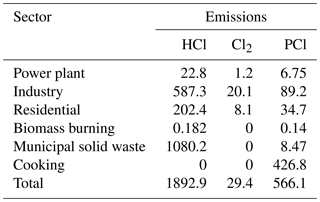Table 2Major gas-phase and heterogeneous pathway of producing nitrate in original CMAQ, and newly added or revised heterogeneous reactions in improved CMAQ.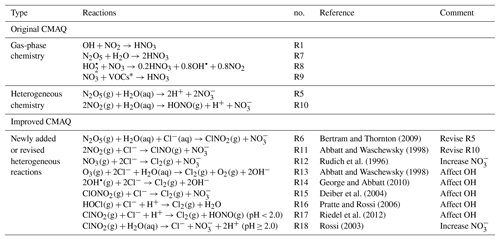Different VOC species. In the SAPRC-11 mechanism, the VOC species include CCHO (acetaldehyde), RCHO (lumped C3+ aldehydes), GLY (glyoxal), MGLY (methylglyoxal), PHEN (phenols), BALD (aromatic aldehydes), MACR (methacrolein) and IPRD (lumped isoprene product species).

## 2.2 Chlorine-related heterogeneous reactions

The heterogeneous reactions in original CMAQ (version 5.0.1) are not related to chlorine species. In this study, the original heterogeneous reactions of N2O5 and NO2 (Reactions R5 and R10 in Table 2) are replaced with a revised version which includes production of ClNO2 from CPs (Reactions R6 and R11 in Table 2). In Reaction (R6), the molar yield of ClNO2 (${\mathit{\varphi }}_{{\mathrm{ClNO}}_{\mathrm{2}}}$) is represented as Eq. (3) (Bertram and Thornton, 2009):

$\begin{array}{}\text{(3)}& {\mathit{\varphi }}_{{\mathrm{ClNO}}_{\mathrm{2}}}={\left(\mathrm{1}+\frac{\left[{\mathrm{H}}_{\mathrm{2}}\mathrm{O}\right]}{\mathrm{483}×\left[{\mathrm{Cl}}^{-}\right]}\right)}^{-\mathrm{1}},\end{array}$

where [H2O] and [Cl] are the molarities of liquid water and chloride (mol m−3), respectively.

In addition, laboratory observations confirmed that the heterogeneous uptake of some oxidants (such as O3 and OH) and reactive chlorine species (such as ClNO2, HOCl and ClONO2) could also occur in CPs to produce Cl2. These reactions are implemented in the model and summarized in Table 2 as Reactions (R13)–(R18). Note that the products from the heterogeneous uptake of ClNO2 in CPs vary with particle acidity (Riedel et al., 2012; Rossi, 2003). It generates Cl2 under the condition of the pH being lower than 2 but produces nitrate and chloride under higher pH conditions. The reaction rates of the heterogeneous reactions are parameterized as first-order reactions, with the rate of change of gas-phase species concentrations determined by Eq. (4) (Ying et al., 2015):

$\begin{array}{}\text{(4)}& \frac{\mathrm{d}C}{\mathrm{d}t}=-\frac{\mathrm{1}}{\mathrm{4}}\left(v\mathit{\gamma }A\right)C=-{k}^{\mathrm{I}}C,\end{array}$

where C represents the concentration of species, v represents the thermal velocity of the gas molecules (m s−1), A is the CMAQ-predicted wet aerosol surface area concentration (m2 m−3) and γ represents the uptake coefficient. For all gas phases, for species (except ClNO2) involved in the heterogeneous reactions (Reactions R6 and R11–R18), a simple analytical solution can be used to update their concentrations from time t0 to t0t: $\left[C{\right]}_{{t}_{\mathrm{0}}+\mathrm{\Delta }t}=\left[C{\right]}_{{t}_{\mathrm{0}}}\mathrm{exp}\left(-{k}^{\mathrm{I}}\mathrm{\Delta }t$), where Δt is the operator-splitting time step for heterogeneous reactions.

The rate of change of ClNO2 includes both removal and production terms, as shown in Eq. (5):

$\begin{array}{ll}\text{(5)}& \frac{\mathrm{d}\left[{\mathrm{ClNO}}_{\mathrm{2}}\right]}{\mathrm{d}t}& =-{k}_{i}^{\mathrm{I}}\left[{\mathrm{ClNO}}_{\mathrm{2}}\right]+{k}_{\mathrm{6}}^{\mathrm{I}}{\mathit{\varphi }}_{{\mathrm{ClNO}}_{\mathrm{2}}}\left[{\mathrm{N}}_{\mathrm{2}}{\mathrm{O}}_{\mathrm{5}}\right]& =-{k}_{i}^{\mathrm{I}}\left[{\mathrm{ClNO}}_{\mathrm{2}}\right]+{k}_{\mathrm{6}}^{\mathrm{I}}{\mathit{\varphi }}_{{\mathrm{ClNO}}_{\mathrm{2}}}{\left[{\mathrm{N}}_{\mathrm{2}}{\mathrm{O}}_{\mathrm{5}}\right]}_{{t}_{\mathrm{0}}}\mathrm{exp}\left(-{k}_{\mathrm{6}}^{\mathrm{I}}t\right).\end{array}$

Assuming that ${\mathit{\varphi }}_{{\mathrm{ClNO}}_{\mathrm{2}}}$ is a constant, an analytical solution for Eq. (5) can be obtained, as shown in Eq. (6):

$\begin{array}{ll}& {\left[{\mathrm{ClNO}}_{\mathrm{2}}\right]}_{{t}_{\mathrm{0}}+\mathrm{\Delta }t}={\left[{\mathrm{ClNO}}_{\mathrm{2}}\right]}_{{t}_{\mathrm{0}}}\mathrm{exp}\left(-{k}_{i}^{\mathrm{I}}\mathrm{\Delta }t\right)\\ \text{(6)}& & \phantom{\rule{0.25em}{0ex}}\phantom{\rule{0.25em}{0ex}}\phantom{\rule{0.25em}{0ex}}+\frac{{k}_{\mathrm{6}}^{\mathrm{I}}{\mathit{\varphi }}_{{\mathrm{ClNO}}_{\mathrm{2}}}{\left[{\mathrm{N}}_{\mathrm{2}}{\mathrm{O}}_{\mathrm{5}}\right]}_{{t}_{\mathrm{0}}}}{{k}_{i}^{\mathrm{I}}-{k}_{\mathrm{6}}^{\mathrm{I}}}\left[\mathrm{exp}\left(-{k}_{\mathrm{6}}^{\mathrm{I}}\mathrm{\Delta }t\right)-\mathrm{exp}\left(-{k}_{i}^{\mathrm{I}}\mathrm{\Delta }t\right)\right],\end{array}$

where ${k}_{i}^{\mathrm{I}}$ represents the pseudo-first-order rate coefficient of either Reaction (R17) or (R18), depending on pH.

The uptake coefficients γ of gaseous species are obtained from published laboratorial studies. In the original CMAQ, the uptake coefficient of N2O5 is determined as a function of the concentrations of (NH4)2SO4, NH4HSO4 and NH4NO3 (Davis et al., 2008). In this study, the parameterization dependent on PCl and ${\mathrm{NO}}_{\mathrm{3}}^{-}$ by Bertram and Thornton (2009) (Eq. 7) is used:

$\begin{array}{ll}\text{(7)}& & {\mathit{\gamma }}_{{\mathrm{N}}_{\mathrm{2}}{\mathrm{O}}_{\mathrm{5}}}=& \phantom{\rule{0.25em}{0ex}}\phantom{\rule{0.25em}{0ex}}\left\{\begin{array}{l}\mathrm{0.02},\phantom{\rule{0.25em}{0ex}}\phantom{\rule{0.25em}{0ex}}\phantom{\rule{0.25em}{0ex}}\text{for\hspace{0.17em} frozen\hspace{0.17em} aerosols}\\ \mathrm{3.2}×{\mathrm{10}}^{-\mathrm{8}}{K}_{\mathrm{f}}\left[\mathrm{1}-{\left(\mathrm{1}+\frac{\mathrm{6}×{\mathrm{10}}^{-\mathrm{2}}\left[{\mathrm{H}}_{\mathrm{2}}\mathrm{O}\right]}{\left[{\mathrm{NO}}_{\mathrm{3}}^{-}\right]}+\frac{\mathrm{29}\left[\mathrm{Cl}\right]}{\left[{\mathrm{NO}}_{\mathrm{3}}^{-}\right]}\right)}^{-\mathrm{1}}\right]\end{array}\right\.\end{array}$

In the above equation, Kf is parameterized function based on the molarity of water: ${K}_{\mathrm{f}}=\mathrm{1.15}×{\mathrm{10}}^{\mathrm{6}}\left(\mathrm{1}-{e}^{-\mathrm{0.13}\left[{\mathrm{H}}_{\mathrm{2}}\mathrm{O}\right]}$). ${\mathrm{NO}}_{\mathrm{3}}^{-}$ and Cl concentrations are also molar. The uptake coefficient of OH is expressed in Eq. (8) as a function of the concentration of PCl following the IUPAC (International Union of Pure and Applied Chemistry, available at http://iupac.poleether.fr/htdocs/datasheets/pdf/O-H_halide_solutions_VI.A2.1.pdf, last access: 5 June 2018).

$\begin{array}{}\text{(8)}& {\mathit{\gamma }}_{\mathrm{OH}}=min\left(\mathrm{0.04}×\frac{\left[{\mathrm{Cl}}^{-}\right]}{\mathrm{1000}×M},\mathrm{1}\right),\end{array}$

where M represents the volume of liquid water in aerosol volume (m3 m−3). For frozen particles, the uptake coefficient is limited to 0.02, as used in the original CMAQ model.

The uptake coefficients of O3, NO3, NO2, HOCl, ClNO2 and ClONO2 are treated as constants. Among of them, the γ values of NO3, NO2, HOCl and ClONO2 are set as $\mathrm{3}×{\mathrm{10}}^{-\mathrm{3}}$, $\mathrm{1}×{\mathrm{10}}^{-\mathrm{4}}$, $\mathrm{1.09}×{\mathrm{10}}^{-\mathrm{3}}$ and 0.16 based on laboratory measurements (Rudich et al., 1996; Abbatt and Waschewsky, 1998; Pratte and Rossi, 2006; Gebel and Finlayson-Pitts, 2001). A preliminary value of 10−3 in the daytime and 10−5 during the nighttime is chosen for the O3 uptake coefficient. The daytime ${\mathit{\gamma }}_{{\mathrm{O}}_{\mathrm{3}}}$ is based on the analysis of the Cl2 production rate in a hypothesized geochemical cycle of reactive inorganic chlorine in the marine boundary layer by Keene et al. (1990). The lower nighttime value was also recommended by Keene et al. (1990), who noted that Cl2 production in the marine boundary layer is lower at night. The uptake coefficient of ClNO2 depends on the particle acidity, with the value of $\mathrm{2.65}×{\mathrm{10}}^{-\mathrm{6}}$ for Reaction (R17) and $\mathrm{6}×{\mathrm{10}}^{-\mathrm{3}}$ for Reaction (R18) (Roberts et al., 2008).

## 2.3 CMAQ model configuration

These heterogeneous reactions of chlorine are incorporated into a revised CMAQ based on the CMAQ version 5.0.1 to simulate the distribution of nitrate concentration in Beijing from 11 to 15 June 2017. The revised CMAQ model without heterogeneous reactions of chlorine has been described in detail by Ying et al. (2015) and Hu et al. (2016, 2017). In summary, the gas-phase chemical mechanism in the revised CMAQ model is based on the SAPRC-11 (Carter and Heo, 2013) with comprehensive inorganic chlorine chemistry. Reactions of the Cl radical with several major VOCs, which lead to production of HCl, are also included. The aerosol module is based on AERO6, with an updated treatment of the NO2 and SO2 heterogeneous reaction and formation of secondary organic aerosol from isoprene epoxides. Three-level nested domains with the resolutions of 36, 12 and 4 km using Lambert conformal conic projection (173×136, 135×228 and 60×66 grid cells) are chosen in this work (the domains; see Fig. S1). The two true latitudes are set to 25 and 40 N, and the origin of the domain is set at 34 N, 110 E. The left-bottom coordinates of the outmost domain are positioned at $x=-\mathrm{3114}$ km, $y=-\mathrm{2448}$ km. The BASE case (heterogeneous reactions of Cl turned off) and HET case (all heterogeneous reactions enabled) are compared to evaluate the impact of heterogeneous chlorine chemistry on nitrate formation.

3 Results

## 3.1 Model performance evaluation

Predicted O3, NO2 and PM2.5 concentrations from the BASE case simulation are evaluated against monitoring data at 12 sites in Beijing (Table S2) in 11 to 15 June 2017. The average values for normalized mean bias (NMB) for O3, NO2 and PM2.5 across the 12 sites are −8 %, −7 % and −8 %, respectively, and 29 %, 59 % and 53 % for normalized mean error (NME), respectively. Predicted hourly Cl2, ClNO2 and N2O5 concentrations were compared with observations measured at the Institute of Atmospheric Physics (IAP), Chinese Academy of Sciences (39.98 N, 116.37 E), using a high-resolution time-of-flight chemical ionization mass spectrometer (CIMS) from 11 to 15 June 2017 (for site description, instrument introduction and analytical method, please refer to the study by Zhou et al., 2018). Figure 1 shows that the concentrations of Cl2 and ClNO2 in the BASE case are rather low (close to 0), proving that the gas-phase chemistry is not the major pathway for producing Cl2 and ClNO2. By contrast, the simulated Cl2 and ClNO2 concentrations in HET case increase significantly; correspondingly the NMB and NME changes from −100 % to −54 % and 100 % to 61 % for Cl2 and from −100 % to −58 % and 100 % to 62 % for ClNO2, respectively (the particle surface area concentrations is scaled up by a factor of 5 during the daytime and 10 during the nighttime because this parameter is underestimated compared to the measured concentrations reported by Zhou et al., 2018). The simulations of Cl2 and ClNO2 are improved as the additional heterogeneous reactions prompt the production of gas-phase molecular chlorine. Overall, however, the Cl2 and ClNO2 concentrations are still underestimated. Both BASE and HET simulations generally capture the hourly N2O5 concentrations as well as the peak values (Fig. 1c) with similar overall NMB and NME values.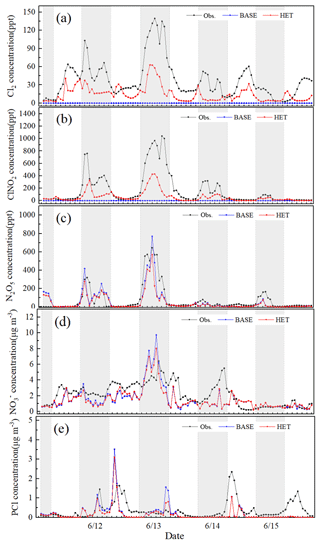Figure 1Comparison of observed hourly Cl2, ClNO2, N2O5 (at the Institute of Atmospheric Physics, Chinese Academy of Sciences), ${\mathrm{NO}}_{\mathrm{3}}^{-}$ and PCl (at Tsinghua University) in urban Beijing with predictions from the BASE and the HET cases during 11–15 June 2017.

Download

The uptake coefficient of O3 could be an important factor affecting the predicted Cl2 concentrations, as the heterogeneous reaction of O3 is found to be the major source of Cl2 during this period (see discussion in Sect. 3.2). The influence of different parametrizations of the uptake coefficient of N2O5 on ClNO2 and nitrate concentrations are also discussed in Sect. 3.2.

Predicted ${\mathrm{NO}}_{\mathrm{3}}^{-}$ and PCl concentrations are compared with observations measured at an adjacent monitoring site located at the rooftop of School of Environment building at Tsinghua University (THU; 40.00 N, 116.34 E; about 5 km from the IAP) using an online analyzer of Monitoring for Aerosol and Gases in ambient Air (MARGA) from 11 to 15 June 2017. According to Fig. 1d, the simulated nitrate concentration is slightly lower than the observations most of the time. From the evening hours of 12 June to the morning hours of 13 June, observed and simulated nitrate concentrations both increase significantly. The NMB and NME values of hourly nitrate for the HET case (−5 % and 39 %, respectively) are slightly lower than those for the BASE case (−10 % and 46 %) during this high-concentration period. The HET case also generally captures the day-to-day variation of PCl concentration and performs better than the BASE case, correspondingly the NMB and NME are reduced from −48 % and 72 % to −37 % and 67 %. The substantial underestimation of PCl in the daytime on 15 June is likely caused by missing local emissions during this period.

## 3.2 Impact of uptake coefficients of O3 and N2O5 on chlorine species and nitrate

The uptake coefficients of O3 and N2O5 may be important factors affecting the accuracy of simulated nitrate concentrations. Some studies have confirmed that the reaction of O3 in CPs can indirectly affect the nitrate formation by increasing the atmospheric Cl2 and OH level (Li et al., 2016; Liu et al., 2018). According to Fig. 1a, the improved model still substantially underestimates the concentration of Cl2, which may be associated with the underestimation of the uptake coefficients of O3, which are empirical and have not been confirmed by laboratory studies. The uptake coefficients were increased by a factor of 10 (0.01 for daytime and 10−4 for nighttime) to evaluate the sensitivity of Cl2 production and nitrate formation to this parameter. Figure 2 shows that the simulated Cl2 and nitrate concentrations in daytime increase significantly (especially for Cl2) and can sometimes capture the peak value (such as the daytime peak on 14 June). However, although the NMB and NME of Cl2 and nitrate improve from −18 % and 39 % to 1 % and 28 % when the new uptake coefficients are used, the simulated Cl2 concentrations are still quite different from the observations (such as during the daytime on 11 and 12 June; see Fig. 2). A non-constant parameterization of the uptake coefficients of O3 that considers the influence of PCl concentrations, meteorology conditions, etc., similar to those of OH and N2O5, might be needed. Further laboratory studies should be conducted to provide a better estimation of this important parameter.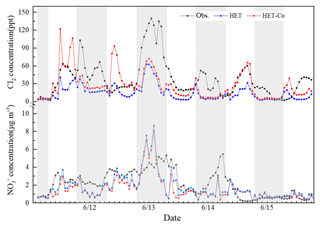Figure 2Comparison of observed and predicted Cl2 and ${\mathrm{NO}}_{\mathrm{3}}^{-}$ concentrations under different uptake coefficient of O3 (HET: daytime is ${\mathit{\gamma }}_{{\mathrm{O}}_{\mathrm{3}}}=\mathrm{1}×{\mathrm{10}}^{-\mathrm{3}}$, nighttime is ${\mathit{\gamma }}_{{\mathrm{O}}_{\mathrm{3}}}=\mathrm{1}×{\mathrm{10}}^{-\mathrm{5}}$; HET-Co: daytime is ${\mathit{\gamma }}_{{\mathrm{O}}_{\mathrm{3}}}=\mathrm{1}×{\mathrm{10}}^{-\mathrm{2}}$, nighttime is ${\mathit{\gamma }}_{{\mathrm{O}}_{\mathrm{3}}}=\mathrm{1}×{\mathrm{10}}^{-\mathrm{4}}$).

Download

Several parameterizations for the uptake coefficient of N2O5 have been developed for regional and global models and have been evaluated in several previous studies (Tham et al., 2018; McDuffie et al., 2018a, b). In addition to the parameterization of Bertram and Thornton (2009) used in the HET case, two additional simulations were performed to assess the impact of the uptake coefficient of N2O5 on nitrate formation. The first simulation uses the original CMAQ parameterization of Davis et al. (2008), and the second simulation uses a constant value of 0.09, which is the upper limit of the N2O5 uptake coefficient derived by Zhou et al. (2018), based on observations. The results from the simulations with the parameterization of Bertram and Thornton (2009) generally agree with the results using those based on Davis et al. (2008) .The application of a larger and fixed N2O5 uptake coefficient leads to slightly better results, which might reflect the fact that the N2O5 concentrations are underestimated. Using the uptake coefficient of 0.09 can generally increase the concentration of nitrate in some periods, but it also leads to significant increase in the nitrate level (such as nighttime on 12–13 June and 13–14 June), which is 4–6 times higher than those based on Bertram and Thornton (2009). Overall, predicted nitrate concentrations are sensitive to changes in the changes in ${\mathit{\gamma }}_{{\mathrm{N}}_{\mathrm{2}}{\mathrm{O}}_{\mathrm{5}}}$, with an increase of approximately 50 % in the nitrate when a constant of ${\mathit{\gamma }}_{{\mathrm{N}}_{\mathrm{2}}{\mathrm{O}}_{\mathrm{5}}}$ of 0.09 is used.

## 3.3 Spatial distributions of nitrate and chlorine species concentrations

The regional distributions of averaged Cl2, ClNO2, N2O5 and ${\mathrm{NO}}_{\mathrm{3}}^{-}$ concentration from 11 to 15 June for the HET case are shown in Fig. 3. Compared to the BASE case, the episode-average concentrations of Cl2 and ClNO2 from the HET case increase significantly in the eastern region of Beijing, reaching up to 23 and 71 ppt from near zero (Fig. 3a and b). High concentrations are not found in the southern region with intensive emissions of chlorine species (Fig. S2). The production of ClNO2 requires the presence of chloride, NO2 and O3. In the areas close to the fresh emissions, O3 is generally low (Fig. S3), and the production of NO3 (hence N2O5 and ClNO2) is limited. Therefore, the production rate of ClNO2 is generally low in areas affected by fresh emissions. Since the contribution of direct emissions to Cl2 is low and it is predominantly produced secondarily in the atmosphere, high levels of Cl2 are also found away from the fresh emissions.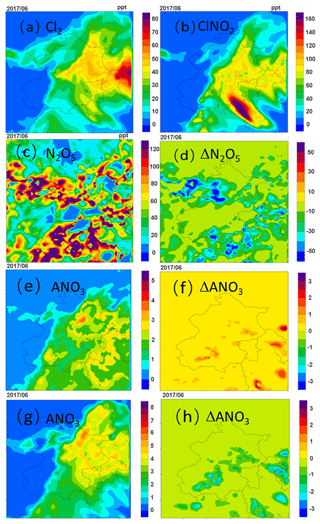Figure 3Spatial distributions of episode-average (a) Cl2, (b) ClNO2, (c) N2O5, (e) daytime nitrate (ANO3) and (g) nighttime nitrate concentrations from 11–15 June 2017, and the differences in the episode-average (d) N2O5 (HET case minus BASE case), (f) daytime nitrate and (g) nighttime nitrate (units are µg m−3).

Download

Table 3Observed day (D) and night (N) ${\mathrm{NO}}_{\mathrm{3}}^{-}$ concentrations (Obs.) and predicted uptake coefficient of N2O5 (${\mathit{\gamma }}_{{\mathrm{N}}_{\mathrm{2}}{\mathrm{O}}_{\mathrm{5}}}$) and nitrate concentrations (Pred.) using the parameterizations of ${\mathit{\gamma }}_{{\mathrm{N}}_{\mathrm{2}}{\mathrm{O}}_{\mathrm{5}}}$ by Bertram and Thornton (2009; Scenario 1), Davis et al. (2008; Scenario 2) and the upper-limit value derived by Zhou et al. (2018; Scenario 3) from 11 to 15 June 2017.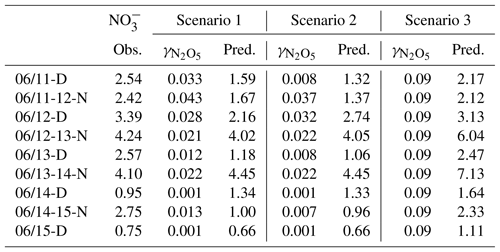The spatial distribution of N2O5 concentrations differs from that of other species (Fig. 3c). While the concentrations of most of the species are higher in the southern region, the N2O5 concentrations are lower in some parts of this region. This is because the O3 concentration in the core urban areas is low due to high NOx emissions. The N2O5 concentrations from the HET case are approximately 16 % lower on average (Fig. 3d) because the Bertram and Thornton (2009) parameterization used in the HET case generally gives higher uptake coefficients than the parameterization of Davis et al. (2008) used in the BASE case (Table 3).

Although the higher uptake coefficients of N2O5 in the HET case facilitate faster conversion of N2O5 to nitrate, the nitrate concentrations do not always increase. During daytime hours, nitrate concentrations in the HET case increase due to higher OH (Fig. 3e and f; for increased OH, see Fig. S4). During the nighttime, in contrast, the nitrate concentration decreases significantly in some regions by about 22 %, mainly due to lower molar yield of nitrate from the N2O5 heterogeneous reaction in the HET case (Fig. 3g and h). Although ClNO2 produced in the N2O5 reaction also produces nitrate through a heterogenous reaction when the particle pH is above 2, which is true for most regions (see Fig. S5), the uptake coefficient of ClNO2 is significantly lower than that of N2O5 (0.01–0.09 for N2O5 and $\mathrm{6}×{\mathrm{10}}^{-\mathrm{3}}$ for ClNO2), leading to an overall decrease in nitrate production. As the ClNO2 production from the heterogeneous reaction leads to less N2O5 conversion to non-relative nitrate, it may change the overall lifetime of NOx and its transport distances. The magnitude of this change and its implications on ozone and PM2.5 in local and downwind areas should be further studied.

## 3.4 Relationship between nitrate formation and chlorine chemistry

Nitrate production from the homogeneous and heterogeneous pathways in Beijing is approximated by the difference in predicted nitrate concentrations between the BASE or HET case and a sensitivity case without heterogenous reactions. Averaging over the 5 d period, approximately 58 % of the nitrate originates from HNO3 gas-to-particle partitioning and 42 % is from heterogeneous reactions (Fig. 4). This conclusion generally agrees with measurements at Peking University (PKU; 52 % from the heterogeneous process and 48 % from HNO3 partitioning) on 4 polluted days (average in September 2016 reported by Wang et al., 2017). Slightly higher contributions of the homogeneous pathway in this study are expected because of high OH concentrations during the day and lower particle surface areas at night.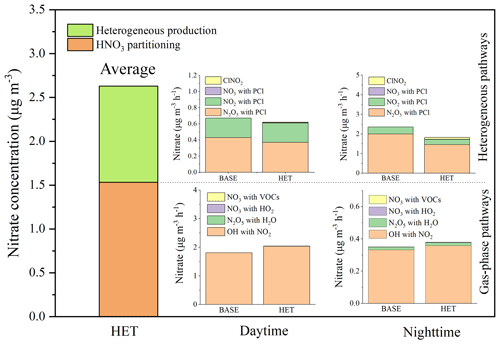Figure 4Contributions of different homogeneous and heterogeneous pathways to nitrate formation.

Download

The nitrate formation from different homogeneous and heterogeneous pathways in the BASE case and HET case are further studied. Contributions of different gas-phase pathways are determined using the process analysis tool in CMAQ. Contributions of different heterogeneous pathways are determined using a zero-out method that turns off one heterogeneous pathway at a time in a series of sensitivity simulations. Figure 4 shows that the reaction of OH and NO2 is always the major pathway for the formation of nitrate through homogeneous formation of HNO3 and gas-to-particle partitioning. However, its nitrate production rate through this homogeneous pathway decreases significantly from daytime to nighttime (from 1.81 to 0.33 µg m−3 h−1 on average). The nitrate production from other HNO3 partitioning pathways in the daytime is negligible. During the nighttime, homogeneous reaction of N2O5 with water vapor accounts for approximately 5 % of the overall homogeneous nitrate formation. For the heterogeneous pathways, daytime production rate is approximately 0.6 µg m−3 h−1, with one-third of the contributions being from NO2 and two-thirds from N2O5. Nighttime production on nitrate from the heterogeneous pathways is approximately 3.1 µg m−3 h−1, of which 85 % is due to N2O5 and 15 % is due to NO2.

Comparing the BASE case and the HET case shows that, when the chlorine chemistry is included, the gaseous HNO3 produced by OH reacting with NO2 increases significantly in the HET case. Correspondingly, the nitrate production rate reaches up to 2.04 µg m−3 h−1 in the daytime due to increased atmospheric OH concentrations predicted by the chlorine reactions. Similar conclusions are also obtained by Li et al. (2016) and Liu et al. (2017) based on observations and model simulations. The heterogeneous production of nitrate from the reaction of N2O5 uptake decreases by approximately 27 % in the HET case due to the production of gas-phase ClNO2. According to the study by Sarwar et al. (2012, 2014), including the heterogeneous reaction of N2O5 with PCl decreased the nocturnal nitrate concentration by 11 %–21 % in the United States, which was slightly less than the current study for Beijing. It is likely because PCl concentrations in the United States are significantly lower than those in Beijing (the monthly PCl concentration is 0.06 µg m−3 in the United States against ∼1µg m−3 in Beijing) so that PCl is depleted quickly. The contributions of NO2 uptake to nitrate also decrease by 22 % because of the lower rate constant of the reaction of NO2 with PCl. In contrast, the contribution of ClNO2 reacts with the particle surface to nitrate production increases of 6 % in the HET case. The overall nitrate concentration in the HET case is about 22 % higher than that in the BASE case during this study period.

4 Conclusions

In this work, a modified CMAQ model incorporating heterogeneous reactions for the production of molecular chlorine and other reactive chlorine species is developed, and its impact on the nitrate formation predictions is evaluated. The contributions from different homogenous and heterogeneous pathways to nitrate formation are also quantified. High concentrations of Cl2 and ClNO2 do not occur in the southern part of the Beijing–Tianjin–Hebei region with intensive emissions of chlorine species, as higher concentrations of O3 and N2O5 associated with the heterogeneous formation of these species generally occurred in the downwind areas. CTMs without a complete treatment of the chlorine chemistry can underestimate daytime nitrate formation from the homogeneous pathways, particularly from HNO3 gas-to-particle partitioning due to underestimation of OH concentrations and overestimation of the nighttime nitrate formation from the heterogeneous pathways due to missing chlorine heterogeneous chemistry.

Data availability

The data in this study are available from the authors upon request (shxwang@tsinghua.edu.cn).

Supplement

The supplement related to this article is available online at: https://doi.org/10.5194/acp-19-6737-2019-supplement.

Author contributions

XQ, QY, SW and JH designed the study. YS, BL, AS and XY provided observation data. XQ, QY, SW, JZ, QX, DD, LD and JX analyzed data. XQ, QY and SW wrote the paper.

Competing interests

The authors declare that they have no conflict of interest.

Acknowledgements

The simulations were completed on the Explorer 100 cluster system of the Tsinghua National Laboratory for Information Science and Technology.

Financial support

This research has been supported by the National Natural Science Foundation of China (grant no. 21625701), the China Postdoctoral Science Foundation (grant no. 2018M641385), the National Research Program for Key Issue in Air Pollution Control (grant nos. DQGG0301, DQGG0501) and the National Key R&D Program of China (grant nos. 2018YFC0213805, 2018YFC0214006).

Review statement

This paper was edited by Andreas Hofzumahaus and reviewed by three anonymous referees.

References

Abbatt, J. P. D. and Waschewsky, G. C.G.: Heterogeneous interactions of HOBr, HNO3, O3, and NO2 with deliquescent NaCl aerosols at room temperature, J. Phys. Chem A., 102, 3719–3725, 1998.

Bertram, T. H. and Thornton, J. A.: Toward a general parameterization of N2O5 reactivity on aqueous particles: the competing effects of particle liquid water, nitrate and chloride, Atmos. Chem. Phys., 9, 8351–8363, https://doi.org/10.5194/acp-9-8351-2009, 2009.

Brown, S. S. and Stutz, J.: Nighttime radical observations and chemistry, Chem. Soc. Rev., 41, 6405–6447, 2012.

Carter, W. P. and Heo, G.: Development of revised SAPRC aromatics mechanisms, Atmos. Environ., 77, 404–414, 2013.

Chang, X., Wang, S., Zhao, B., Cai, S., and Hao, J.: Assessment of inter-city transport of particulate matter in the Beijing–Tianjin–Hebei region, Atmos. Chem. Phys., 18, 4843–4858, https://doi.org/10.5194/acp-18-4843-2018, 2018.

Davis, J. M., Bhave, P. V., and Foley, K. M.: Parameterization of N2O5 reaction probabilities on the surface of particles containing ammonium, sulfate, and nitrate, Atmos. Chem. Phys., 8, 5295–5311, https://doi.org/10.5194/acp-8-5295-2008, 2008.

Deiber, G., George, Ch., Le Calvé, S., Schweitzer, F., and Mirabel, Ph.: Uptake study of ClONO2 and BrONO2 by Halide containing droplets, Atmos. Chem. Phys., 4, 1291–1299, https://doi.org/10.5194/acp-4-1291-2004, 2004.

Deng, S., Zhang, C., Liu, Y., Cao, Q., Xu, Y. Y., Wang, H. L., and Zhang, F.: A Full-Scale Field Study on Chlorine Emission of Pulverized Coal-Fired Power Plants in China, Research of Environmental Science, 27, 127–133, 2014 (in Chinese).

Faxon, C. B., Bean, J. K., and Hildebrandt R. L.: Inland Concentrations of Cl2 and ClNO2 in Southeast Texas Suggest Chlorine Chemistry Significantly Contributes to Atmospheric Reactivity, Atmosphere, 6, 1487–1506, 2015.

Fu, X., Wang, S. X., Chang, X., Xing, J., and Hao, J. M.: Modeling analysis of secondary inorganic aerosols over China: pollution characteristics, and meteorological and dust impacts, Sci. Rep., 6, 35992, https://doi.org/10.1038/srep35992, 2016.

Fu, X., Wang, T., Wang, S. X., Zhang, Lei, Cai, S. Y., Xing, J., and Hao, J. M.: Anthropogenic Emissions of Hydrogen Chloride and Fine Particulate Chloride in China, Environ. Sci. Technol., 52, 1644–1654, 2018.

Gebel, M. E. and Finlayson-Pitts, B. J.: Uptake and reaction of ClONO2 on NaCl and synthetic sea salt, J. Phys. Chem. A, 105, 5178–5187, 2001.

George, I. J. and Abbatt, J. P.: Heterogeneous oxidation of atmospheric aerosol particles by gas-phase radicals, Nat. Chem. 2, 713–722, 2010.

Glasow, V. R.: Wider role for airborne chlorine, Nature, 464, 168–169, 2010.

Goodman, A. L., Underwood, G. M., and Grassian, V. H.: Heterogeneous reaction of NO2: characterization of gas-phase and adsorbed products from the reaction, 2${\mathrm{NO}}_{\mathrm{2}}\left(\mathrm{g}\right)+{\mathrm{H}}_{\mathrm{2}}\mathrm{O}\left(\mathrm{a}\right)\to \mathrm{HONO}\left(\mathrm{g}\right)+{\mathrm{HNO}}_{\mathrm{3}}\left(\mathrm{a}\right)$ on Hydrated Silica Particles, J. Phys. Chem. A, 103, 7217–7223, 1999.

Hu, J., Chen, J., Ying, Q., and Zhang, H.: One-year simulation of ozone and particulate matter in China using WRF/CMAQ modeling system, Atmos. Chem. Phys., 16, 10333–10350, https://doi.org/10.5194/acp-16-10333-2016, 2016.

Hu, J., Wang, P., Ying, Q., Zhang, H., Chen, J., Ge, X., Li, X., Jiang, J., Wang, S., Zhang, J., Zhao, Y., and Zhang, Y.: Modeling biogenic and anthropogenic secondary organic aerosol in China, Atmos. Chem. Phys., 17, 77–92, https://doi.org/10.5194/acp-17-77-2017, 2017.

Jobson, B. T., Niki, H., Yokouchi, Y., Bottenheim, J. W., Hopper, F., and Leaitch, R. J.: Measurements of C2–C6 hydrocarbons during the Polar Sunrise 1992 experiment: Evidence for Cl atom and Br atom chemistry, J. Geophys. Res., 99, 25355–25368, 1994.

Keene, W. C., Pszenny, A. A. P., Jacob, D. J., Duce, R. A., Galloway, J. N., Schultz-Tokos, J. J., Sievering, H., and Boatman, J. F.: The Geochemical Cycling of Reactive Chlorine through the Marine Troposphere, Global Biogeochem., 4, 407–430, 1990.

Keene, W. C., Khalil, M. A. K., Erickson, D. J., McCulloch, A., Graedel, T. E., Lobert, J. M., Aucott, M. L., Gong, S. L., Harper, D. B., Kleiman, G., Midgley, P., Moore, R. M., Seuzaret, C., Sturges, W. T., Benkovitz, C. M., Koropalov, V., Barrie, L., and Li, Y. F.: Composite global emissions of reactive chlorine from anthropogenic and natural sources: Reactive Chlorine Emissions Inventory, J. Geophys. Res.-Atmos., 104, 8429–8440, 1999.

Kleeman, M. J., Ying, Q., and Kaduwela, A.: Control strategies for the reduction of airborne particulate nitrate in California's San Joaquin Valley, Atmos. Environ., 39, 5325–5341, 2005.

Knipping, E. M., Lakin, M. J., Foster, K. L., Jungwirth, P., Tobias, D. J., Gerber, R. B., Dabdub, D., and Finlayson-Pitts, B. J.: Experiments and simulations of ion-enhanced interfacial chemistry on aqueous NaCl aerosols, Science, 288, 301–306, 2000.

Li, H., Zhang, Q., Zheng, B., Chen, C., Wu, N., Guo, H., Zhang, Y., Zheng, Y., Li, X., and He, K.: Nitrate-driven urban haze pollution during summertime over the North China Plain, Atmos. Chem. Phys., 18, 5293–5306, https://doi.org/10.5194/acp-18-5293-2018, 2018.

Li, Q., Zhang, L., Wang, T., Tham, Y. J., Ahmadov, R., Xue, L., Zhang, Q., and Zheng, J.: Impacts of heterogeneous uptake of dinitrogen pentoxide and chlorine activation on ozone and reactive nitrogen partitioning: improvement and application of the WRF-Chem model in southern China, Atmos. Chem. Phys., 16, 14875–14890, https://doi.org/10.5194/acp-16-14875-2016, 2016.

Liu, X. X., Qu, H., Huey, L. G., Wang, Y. W., Sjostedt, S., Zeng, L. M., Lu, K. D., Wu, Y. S., Hu, M., Shao, M., Zhu, T., and Zhang, Y. H.: High Levels of Daytime Molecular Chlorine and Nitryl Chloride at a Rural Site on the North China Plain, Environ. Sci. Technol., 51, 9588–9595, 2017.

Liu, Y., Fan, Q., Chen, X., Zhao, J., Ling, Z., Hong, Y., Li, W., Chen, X., Wang, M., and Wei, X.: Modeling the impact of chlorine emissions from coal combustion and prescribed waste incineration on tropospheric ozone formation in China, Atmos. Chem. Phys., 18, 2709–2724, https://doi.org/10.5194/acp-18-2709-2018, 2018.

Ma, X. Y., Sha, T., Wang, J. Y., Jia, H. L., and Tian, R.: Investigating impact of emission inventories on PM2.5 simulations over North China Plain by WRF-Chem, Atmos. Environ., 195, 125–140, 2018.

McDuffie, E. E., Fibiger, D. L., Dubé, W. P., Hilfiker, F., Lee, B. H., Thornton, J. A., Shah, V., Jaeglé, V., Guo, H. Y., Weber, R. J., Reeves, J. M., Weinheimer, A. J., Schroder, C. Campuzano-Jost, P., Jimenez, J., Dibb, J. E., Veres, P., Ebben, C., Sparks, T. L., Wooldridge, P. J., Cohen, R. C., Hornbrook, R. S., Apel, E. C., Campos, T., Hall, S. R., Ullmann, K., and Brown, S. S.: Heterogeneous N2O5 uptake during winter: Aircraft measurements during the 2015 WINTER campaign and critical evaluation of current parameterizations, J. Geophys. Res.-Atmos., 123, 4345–4372, 2018a.

McDuffie, E. E., Fibiger, D. L., Dubé, W. P., Hilfiker, F., Lee, B. H., Thornton, J. A., Shah, V., Jaeglé, V., Guo, H. Y., Weber, R. J., Reeves, J. M., Weinheimer, A. J., Schroder, C. Campuzano-Jost, P., Jimenez, J., Dibb, J. E., Veres, P., Ebben, C., Sparks, T. L., Wooldridge, P. J., Cohen, R. C., Hornbrook, R. S., Apel, E. C., Campos, T., Hall, S. R., Ullmann, K., and Brown, S. S.: ClNO2 yields from aircraft measurements during the 2015 WINTER campaign and critical evaluation of the current parameterization, J. Geophys.Res.-Atmos., 123, 12–994, 2018b.

Osthoff, H. D., Roberts, J. M., and Ravishankara, A. R.: High levels of nitryl chloride in the polluted subtropical marine boundary layer, Nat. Geosci., 1, 324–328, 2008.

Pratte, P. and Rossi, M. J.: The heterogeneous kinetics of HOBr and HOCl on acidified sea salt and model aerosol at 40–90 % relative humidity and ambient temperature, Phys. Chem. Chem. Phys., 8, 3988–4001, 2006.

Qiu, X. H., Chai, F. H., Duan, L., Chai, F. H., Wang, S. X., Yu, Q., and Wang, S. L.: Deriving High-Resolution Emission Inventory of Open Biomass Burning in China based on Satellite Observations, Environ. Sci. Technol., 50, 11779–11786, 2016.

Riedel, T. P., Bertram, T. H., Crisp, T. A., Williams, E. J., Lerner, B. M., Vlasenko, A., Li, S. M., Gilman, J., de Gouw, J., Bon, D. M., Wagner, N. L., Brown, S. S., and Thornton, J. A.: Nitryl Chloride and Molecular Chlorine in the Coastal Marine Boundary Layer, Environ Sci Technol., 46, 10463–10470, 2012.

Roberts, J. M., Osthoff, H. D., Brown, S. S., and Ravishankara, A. R.: N2O5 oxidizes chloride to Cl2 in acidic atmospheric aerosol, Science, 321, 1059–1059, 2008.

Rossi, M. J.: Heterogeneous Reactions on Salts, Chem. Rev., 103, 4823–4882, 2003.

Rudich, Y., Talukdar, R. K., Ravishankara, A. R., and Fox, R. W.: Reactive uptake of NO3 on pure water and ionic solutions, J. Geophys. Res., 101, 21023–21031, 1996.

Sarwar, G., Simon, H., Bhave, P., and Yarwood, G.: Examining the impact of heterogeneous nitryl chloride production on air quality across the United States, Atmos. Chem. Phys., 12, 6455–6473, https://doi.org/10.5194/acp-12-6455-2012, 2012.

Sarwar, G., Simon, H., and Xing, J: Importance of tropospheric ClNO2 chemistry across the Northern Hemisphere, Geophys. Res. Lett., 41, 4050–4058, 2014.

Seinfeld, J. H. and Pandis, S. N.: Atmospheric Chemistry and Physics: From Air Pollution to Climate Change, Wiley-Interscience, New York, USA, 2006.

Spicer, C. W., Chapman, E. G., Finlayson-Pitts, B. J., Plastridge, R. A., Hubbe, J. M., Fast, J. D., and Berkowitz, C. M.: Unexpectedly high concentrations of molecular chlorine in coastal air, Nature, 394, 353–356, 1998.

Tham, Y. J., Wang, Z., Li, Q., Wang, W., Wang, X., Lu, K., Ma, N., Yan, C., Kecorius, S., Wiedensohler, A., Zhang, Y., and Wang, T.: Heterogeneous N2O5 uptake coefficient and production yield of ClNO2 in polluted northern China: roles of aerosol water content and chemical composition, Atmos. Chem. Phys., 18, 13155–13171, https://doi.org/10.5194/acp-18-13155-2018, 2018.

Thornton, J. A., Kercher, J. P., Riedel, T. P., Wagner, N. L., Cozic, J., Holloway, J. S., Dubé, W. P., Wolfe, G. M., Quinn, P. K., Middlebrook, A. M., Alexander, B., and Brown, S. S.: A large atomic chlorine source inferred from mid-continental reactive nitrogen chemistry, Nature, 464, 271–274, 2010.

Tuazon, E. C., Atkinson, R., Plum, C. N., Winer, A. M., and Pitts Jr., J. N.: The reaction of gas phase N2O5 with water vapor, Geophys. Res. Lett., 10, 953–956, 1983.

Wang, H. C., Lu, K. D., Chen, X. R., Guo. S., Jiang, M. Q., Li, X., Shang, D. J., Tian, Z. F., Wu, Y. S., Wu, Z. J., Zou, Q., Zheng, Y., Zeng, L. M., Zhu, T. Hu, M., and Zhang, Y. H.: High N2O5 concentrations observed in urban Beijing: implications of a large nitrate formation pathway, Environ. Sci. Technol. Lett., 4, 416–420, 2017.

Wang, S. X., Zhao, B., Cai, S. Y., Klimont, Z., Nielsen, C. P., Morikawa, T., Woo, J. H., Kim, Y., Fu, X., Xu, J. Y., Hao, J. M., and He, K. B.: Emission trends and mitigation options for air pollutants in East Asia, Atmos. Chem. Phys., 14, 6571–6603, https://doi.org/10.5194/acp-14-6571-2014, 2014.

Wen, L., Xue, L., Wang, X., Xu, C., Chen, T., Yang, L., Wang, T., Zhang, Q., and Wang, W.: Summertime fine particulate nitrate pollution in the North China Plain: increasing trends, formation mechanisms and implications for control policy, Atmos. Chem. Phys., 18, 11261–11275, https://doi.org/10.5194/acp-18-11261-2018, 2018.

Wu, X. W., Chen, W. W., Wang, K., Xiu, A. J., Zhang, S. C., Zhao, H. M., and Zhang, X. L.: PM2.5 and VOCs emission inventories from cooking in Changchun city, China Environmental Science, 38, 2882–2889, 2018.

Ying, Q., Li, J. Y., and Kota, S. H.: Significant Contributions of Isoprene to Summertime Secondary Organic Aerosol in Eastern United States, Environ. Sci. Technol., 49, 7834–7842, 2015.

Young, C. J., Washenfelder, R. A., Edwards, P. M., Parrish, D. D., Gilman, J. B., Kuster, W. C., Mielke, L. H., Osthoff, H. D., Tsai, C., Pikelnaya, O., Stutz, J., Veres, P. R., Roberts, J. M., Griffith, S., Dusanter, S., Stevens, P. S., Flynn, J., Grossberg, N., Lefer, B., Holloway, J. S., Peischl, J., Ryerson, T. B., Atlas, E. L., Blake, D. R., and Brown, S. S.: Chlorine as a primary radical: evaluation of methods to understand its role in initiation of oxidative cycles, Atmos. Chem. Phys., 14, 3427–3440, https://doi.org/10.5194/acp-14-3427-2014, 2014.

Zhang, T., Peng, L., and Li, Y. H.: Chemical characteristics of PM2.5 emitted from cooking fumes, Res. Environ. Sci., 29, 183–191, 2016 (in Chinese).

Zhou, W., Zhao, J., Ouyang, B., Mehra, A., Xu, W., Wang, Y., Bannan, T. J., Worrall, S. D., Priestley, M., Bacak, A., Chen, Q., Xie, C., Wang, Q., Wang, J., Du, W., Zhang, Y., Ge, X., Ye, P., Lee, J. D., Fu, P., Wang, Z., Worsnop, D., Jones, R., Percival, C. J., Coe, H., and Sun, Y.: Production of N2O5 and ClNO2 in summer in urban Beijing, China, Atmos. Chem. Phys., 18, 11581–11597, https://doi.org/10.5194/acp-18-11581-2018, 2018.